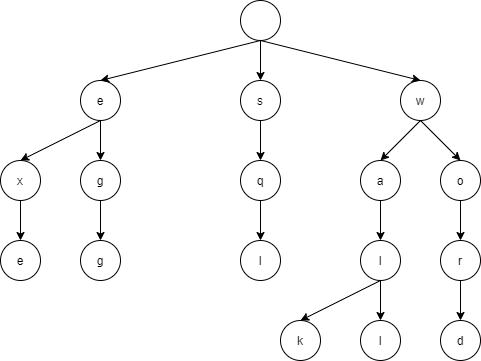# Java implementation of trie tree

## What is a try tree?A trie tree is a type of ordered tree in which each node stores a value of a character, and the algorithm is such that a search can be performed by tracing the nodes.

## Try writing in Java

Create a class that represents a node

``````public class Node {
private int id;
private String str;
private ArrayList<Node> child = new ArrayList<>();
public int getId() {
return this.id;
}
public String getStr() {
return this.str;
}
public ArrayList<Node> getChild(){
return this.child;
}
public void setId(int id) {
this.id = id;
}
public void setStr(String str) {
this.str = str;
}
public void setChild(Node node) {
}
}
``````

id identifies the node and does not have to be str is the character to store in the node child stores the child of the node

The tree can be constructed as follows

``````public class Main {
private static ArrayList<Node> nodeList = new ArrayList<>();
private static int id = 1;
public static void insert(Node parent, String word, int charIndex) {
String w = String.valueOf(word.charAt(charIndex));
//System.out.println(w);
if(charIndex == word.length()-1) {/ if last character
for(Node n:parent.getChild()) {// if the last node is an existing node
if(n.getStr() != null&&n.getStr().equals(w)) {
return;
}
}
Node node = new Node();
node.setStr(w);
parent.setChild(node);
node.setChild(node);
node.setId(id);
id += 1;
return;
}
for(Node n:parent.getChild()) {// if the child contains the current word
if(n.getStr() != null&&n.getStr().equals(w)) {
insert(n,word,charIndex+1);
return;
}
}
Node node = new Node();
node.setStr(w);
parent.setChild(node);
node.setId(id);
id += 1;
if(charIndex <word.length()-1) {// recursive processing
insert(node, word, charIndex+1);
}
}
``````

I am rewriting for the article that I wrote when I was making another algorithm with a little modification of the trie tree, so I am sorry if it is wrong (__)

## Summary

This time, I had an opportunity to use a try tree, so I tried to implement it somehow in java, but it took time to understand because it uses recursive processing. After all, I am not good at recursive processing, so I want to use it aggressively to eliminate my weakness…

Tags:

Updated: